Algebra II : Range

Example Questions

← Previous 1 3 4 5 6 7 8 9 10

Example Question #23 : Basic Statistics

Suppose the ages of a college chemistry class were recorded and the ages reported were as follows:What is the range of this data?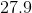Explanation:

The range is calculated by subtracting the smallest value from the largest value: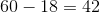Example Question #71 : Basic Statistics

Observe the following table:

 Client Age Marsha 14 Tom 13 Alice 65 Brandy 34 Candy 23 James 43 Brady 19

Find the range for the ages of the clients in the table?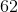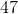Explanation:

Let us start by identifying the maximum and minimum values of the list of values.

The maximum is 65 and the minimum is 13.

The range is computed by substracting the minimum from the maximum, which gives us: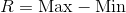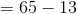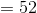Example Question #1 : Data Properties

Observe the following list of terms: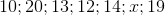The range of this list of numbers is. Which value cantake?Explanation:

The range of this list of numbers is 10 which is obtained by doing 20-10.

This means that 20 and 10 are respectively the maximum and minimum values of the list of numbers.

Therefore x has to be a value between 10 and 20.

17 being the only value within this range it is therefore the right answer.

Example Question #2 : Data Properties

For a set of numbers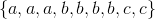where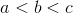and the set has the following values: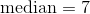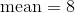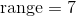Determine the value of.Explanation:

{6,6,6,7,7,7,7,13,13}

median=7 so b=7

mean=8 so the sum of the set is 72.

72-4(7)=44

44=3a+2c

Range=7  so c-a=7

44=3a+2(a+7)

a=6 and c=13.

Example Question #33 : Basic Statistics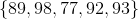Given the set of test scores, what is the range?Explanation:

The range is the difference between the smallest number and the largest number. The smallest value is 77, and the largest is 98.

98 – 77 = 21

Example Question #34 : Basic Statistics

The range of the following data set is 18. What is a possible value for?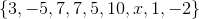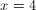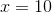Cannot be determined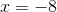Explanation:

Arrange the known values in the set in numerical order: {–5, –2, 1, 3, 5, 7, 7, 10}. The range is the difference between the largest value and smallest value.

x must be either the largest or the smallest value in the set.

range = x – smallest value

18 = x – (–5)

18 = x + 5

13 = x

OR

range = largest value – x

18 = 10 – x

8 = –x

–8 = x

Example Question #1 : How To Find Range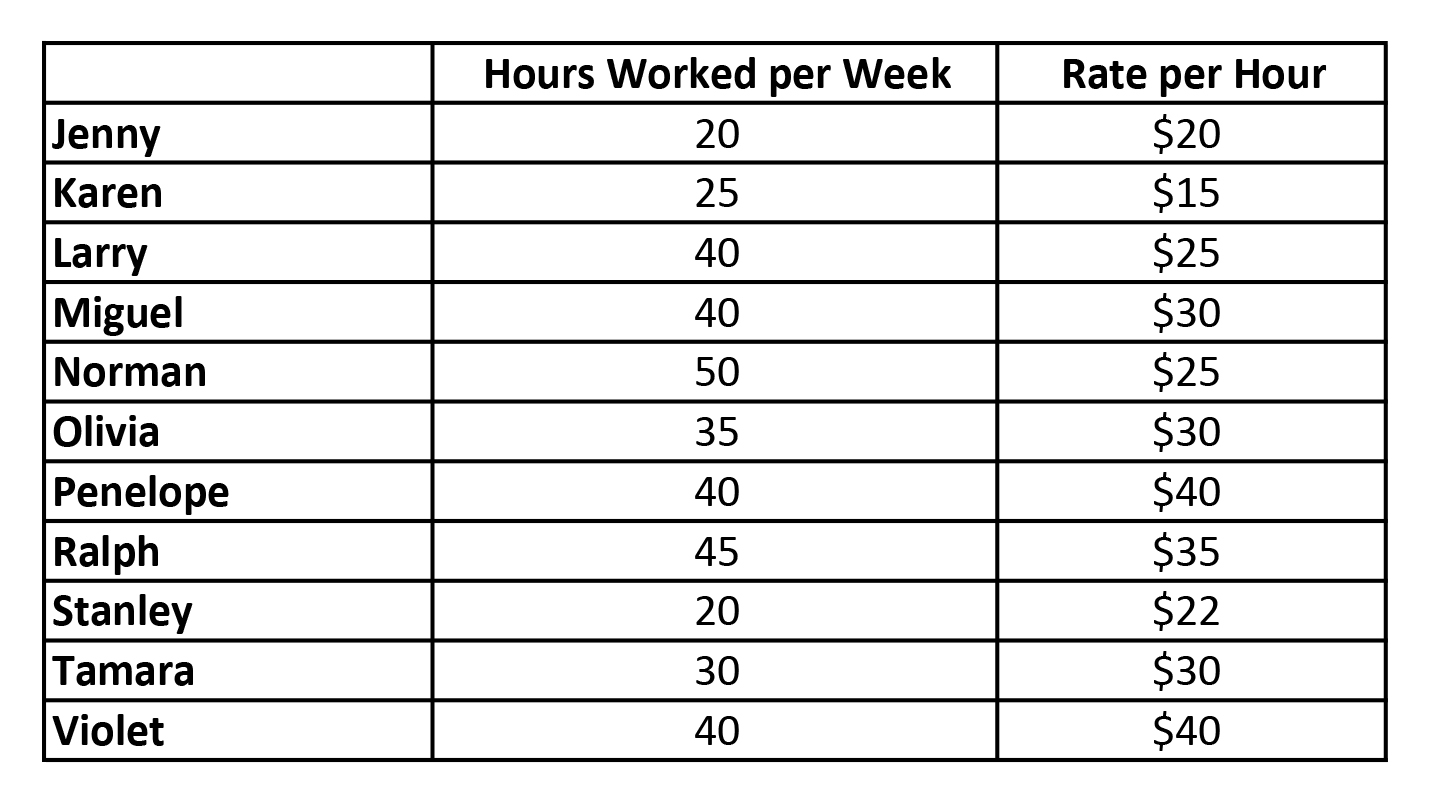The above chart shows a specific week of work at an advertising firm.  What is the range of the hourly rates of the workers?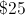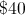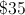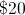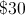Explanation:

The range is difference between the largest value and the smallest value.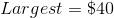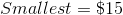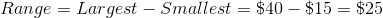Example Question #1 : How To Find Range

What is the range of the set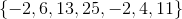?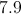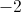Explanation:

The range is defined as the difference between the highest and lowest numbers in a set. Here, the highest number isand the lowest isTherefore, the range is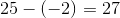is the mode,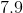is the mean, and 6 is the median.

Example Question #37 : Basic Statistics

Find the range of the set: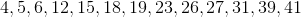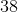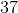Explanation:

To find the range of a set subtract the smallest number in the set from the largest number in the set: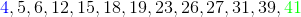The largest number is in green: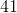The smallest number is in blue:Therefore the range is as follows.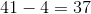Example Question #38 : Basic Statistics

Find the range of the set: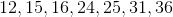Explanation:

To find the range of a set subtract the smallest number in the set from the largest number in the set: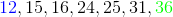The largest number is in green:The smallest number is in blue:Therefore the range is,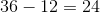.

← Previous 1 3 4 5 6 7 8 9 10

All Algebra II Resources